# Civil Engineering - RCC Structures Design

### Exercise :: RCC Structures Design - Section 1

46.

If T and R are tread and rise respectively of a stair, then

 A. 2R + T = 60 B. R + 2T = 60 C. 2R + T = 30 D. R + 2T= 30 E. 3R + 27 = 30

Explanation:

No answer description available for this question. Let us discuss.

47.

If k is wobble correction factor, μ is coefficient of friction between the duct surface and the curve of tendon of radius R, the tension ratio at a distance x from either end, is

 A.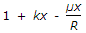B.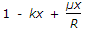C.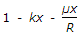D.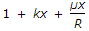E.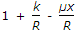Explanation:

No answer description available for this question. Let us discuss.

48.

In a prestressed beam carrying an external load W with a bent tendon is having angle of inclination θ and prestressed load P. The net downward load at the centre is

 A. W - 2P cos θ B. W - P cos θ C. W - P sin θ D. W - 2P sin θ E. W + 2P sin θ

Explanation:

No answer description available for this question. Let us discuss.

49.

The effective width of a column strip of a flat slab, is

 A. one-fourth the width of the panel B. half the width of the panel C. radius of the column D. diameter of the column E. none of these.

Explanation:

No answer description available for this question. Let us discuss.

50.

High strength concrete is used in prestressed member

 A. to overcome high bearing stresses developed at the ends B. to ovecome bursting stresses at the ends C. to provide high bond stresses D. to overcome cracks due to shrinkage E. all the above.